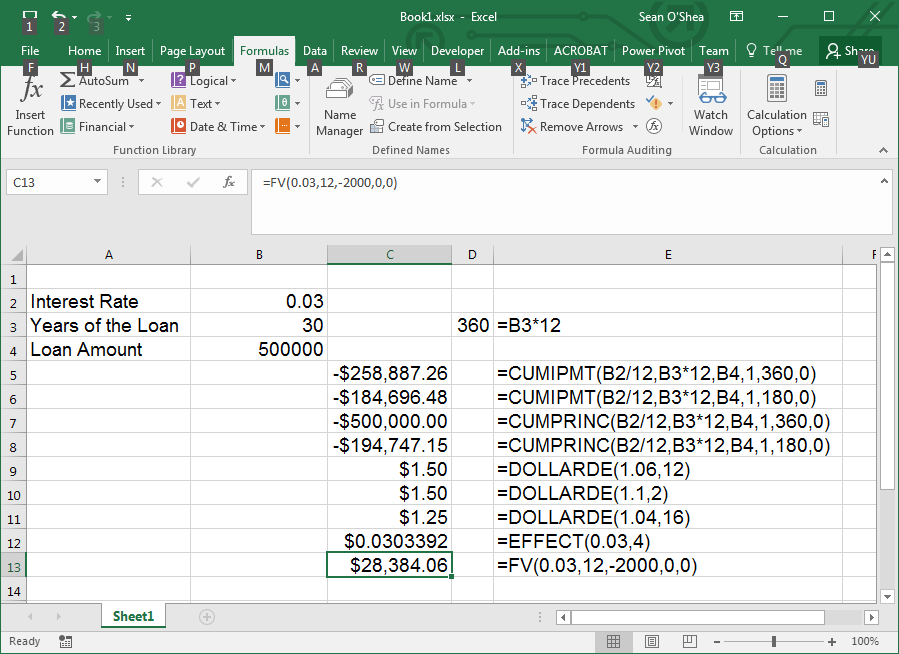Search

# Excel Financial Formulas

Here's a brief rundown of some financial formulas available in Microsoft Excel.

In the screen grab below column E shows the text of the formulas listed in column C.The CUMIPMT formula calculates the total interest paid on a loan between two periods:

=CUMIPMT(Interest Rate/12,Length of Loans in Years * 12,Loan Amount,First Period,Last Period,[0 if the payment is made at the end of the period, 1 if the payment is made at the beginning]).

=CUMIPMT(.03/12,30*12,500000,1,360,0)The CUMPRINC formula calculates the amount of a principal paid between two different periods.

=CUMPRINC(Interest Rate/12,Years of Loan*12,Loan Amount,First Period, Last Period,[0 if the payment is made at the end of the period, 1 if the payment is made at the beginning]).

=CUMPRINC(B2/12,B3*12,B4,1,360,0)The DOLLARDE formula converts a fraction of a dollar into a decimal amount. For example:

=DOLLARDE(1.1,2)

. . . will find the the decimal amount for 1 over 2, or .5.

=DOLLARDE(1.04,16)

. . . will find the decimal amount for 4 over 16, or .25.The EFFECT formula shows the effective annual interest rate based on the number of times interest is compounded. The compounding period is the number of times that unpaid mortgage interest is added to the principal amount of the loan.

=EFFECT(Interest Rate,Compounding Periods)

=EFFECT(0.03,4)The FV formula calculates the total value of an annuity.

=FV(Interest Rate per Period,Number of Payments,Payment in each Period,Present Value,[0 if the payment is made at the end of the period, 1 if the payment is made at the beginning]).